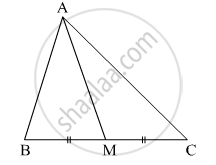Share

# In ∆Abc, Point M is the Midpoint of Side Bc. If, Ab2 + Ac2 = 290 Cm2, Am = 8 Cm, Find Bc. - Geometry

ConceptApollonius Theorem

#### Question

In ∆ABC, point M is the midpoint of side BC.
If, AB+ AC= 290 cm2, AM = 8 cm, find BC.#### Solution

In ∆ABC, point M is the midpoint of side BC.

$BM = MC = \frac{1}{2}BC$

${AB}^2 + {AC}^2 = 2 {AM}^2 + 2 {BM}^2 \left( \text{by Apollonius theorem} \right)$
$\Rightarrow 290 = 2 \left( 8 \right)^2 + 2 {BM}^2$
$\Rightarrow 290 = 2\left( 64 \right) + 2 {BM}^2$
$\Rightarrow 290 = 128 + 2 {BM}^2$
$\Rightarrow 2 {BM}^2 = 290 - 128$
$\Rightarrow 2 {BM}^2 = 162$
$\Rightarrow {BM}^2 = 81$
$\Rightarrow BM = 9$
$\therefore BC = 2 \times BM$
$= 2 \times 9$
$= 18 cm$

Hence, BC = 18 cm.

Is there an error in this question or solution?

#### APPEARS IN

Solution In ∆Abc, Point M is the Midpoint of Side Bc. If, Ab2 + Ac2 = 290 Cm2, Am = 8 Cm, Find Bc. Concept: Apollonius Theorem.
S